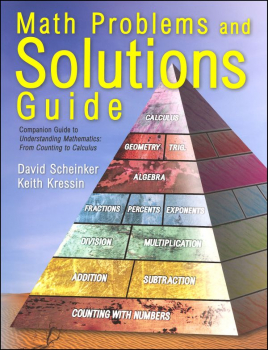# Math Problems and Solutions Guide

# BM-047522

Bargain Price: \$18.43
Retail: \$24.95
Save: 26.13% (\$6.52)
No more Bargain Versions available to purchase.#### Product Description:

A non-technical book that explains math from counting to calculus. Topics build on one another, providing the reader with a complete overview of mathematics. This book is divided into three sections-- elementary (numbers, addition, subtraction, multiplication, division, fractions, percentages, negative numbers, exponents, pre-algebra), intermediate (solving one algebraic equation, solving a system of equations, word problems, functions, graphing, geometry, measurement of geometric figures, trigonometry), and advanced (logarithms, complex numbers, finding geometry, using algebra, introducing calculus, the derivative, the integral). This is not a text, but a discussion that includes numerous worked examples. For parents, students, or anyone who wants to understand math. Also useful as a comprehensive math reference.

It does no good to have a text without practice of the concepts. Students read the content found in the main text "Understanding Mathematics," then complete the corresponding section in the solutions (companion) guide. The first half of the guide offers hints, examples, explanations, exercises, and problems for practice. Each chapter's content builds on the previous chapter, and initial problems incorporate the new concept presented in the main text and more challenging problems with multiple concepts by the end of the chapter. The second half of the book gives the solutions for all of the problems in the book. A 20-question chapter summary concludes each chapter. The text along with the solutions guide provide extra practice in specific skill areas, allow students to work ahead of their curriculum for an added challenge, or can be used for a comprehensive review before taking a standardized test. sc. ~ Ahn/Donna

Primary Subject
Mathematics
6
ISBN
9780965730037
Format
Softcover Book
Brand Name
K Squared Publishing
Weight
1.95 (lbs.)
Dimensions
11.0" x 8.5" x 0.75"